# Event Prediction with the gestate Package

## 1 Introduction

### 1.1 Vignette Overview

The gestate package is designed to assist accurate planning and event prediction for time-to-event clinical trials. In this vignette, we introduce event prediction in the gestate package. A separate vignette (“trial_planning”) introduces gestate and its trial planning tools.

Section 2 will look at the two available methods for fitting parametric curves to existing data. Section 3 will then look at event prediction using these techniques.

### 1.2 Event Prediction

Event prediction is an important aspect of trial conduct for time-to-event trials since analysis time is ultimately linked to the number of events occurring, which is in turn is closely related to power. In cases where performing the analysis is triggered by a set event number, the connection is obvious, but even in trials where there is a fixed assessment time, overestimation of event numbers can lead to a severe loss of power and in these cases there will often be pressure to extend trial length. It is therefore very common to either want to know the number of events that will occur at a given time, or the time at which a given number of events will occur.

### 1.3 Overview of gestate’s scope

The gestate package supports event prediction based upon either patient-level data, or summarised life-table data. It performs parametric modelling using, Weibull, exponential or Log-normal distributions, and in cases where the approximate distribution is not known, can automatically choose the best fit between Weibull or Log-normal. As it is based upon Curve architecture, it also supports arbitrary censoring and recruitment distributions, allowing for flexibility of assumptions. Both conditional and unconditional prediction are included. Where patient-level data is supplied, it will also produce analytic prediction intervals.

## 2 Curve-Fitting

Fitting parametric distributions to a Survival curve is useful for both event prediction and for gathering trial planning assumptions. The gestate package supports curve fitting for Weibull, exponential and Log-normal distributions for both life-table data (fit_KM) patient-level data (fit_tte_data).

### 2.1 Example Data Set

In this section, we will use the following randomly-generated data set, created by simulate_trials, which assumes an underlying Weibull distribution with parameters alpha = 100, beta = 0.8. For more details on this step, see section 4.1 in the vignette on trial planning (“trial_planning”). The second section of this code generates a 7-day interval lifetable; this is to create a data set that can be used to illustrate the use of fit_km, which operates on life-table data. Typically, if you have patient-level data available it is recommended that you should use that directly instead.

library(gestate)
library(survival)
# Simulate a single data set based upon a Weibull curve with shape 0.8 and scale 100:
# Distributions
example_distribution <- Weibull(alpha=100,beta=0.8)
example_recruitment  <- LinearR(rlength=24,Nactive=600,Ncontrol=0)
# Create the full trajectory
maxtime <- 100
example_data_long <- simulate_trials(active_ecurve=example_distribution,control_ecurve=example_distribution,rcurve=example_recruitment,assess=maxtime,iterations=1,seed=1234567,detailed_output=TRUE)
# Shrink the trajectory to an early time point at which event prediction will occur
example_data_short <- set_assess_time(example_data_long,18)

######################
# Create life-table with 7-day 'sampling'
# Note that this is to create an example lifetable and would not normally be done as it is typically better to use patient-level data if available
temp1  <- summary(survfit(Surv(example_data_short[,"Time"],1-example_data_short[,"Censored"])~ 1,error="greenwood"))
out1 <- cbind(temp1$time,temp1$n.risk,temp1$surv,temp1$std.err)
out1 <- rbind(c(0,out1[1,2],1,0),out1)
colnames(out1) <- c("Time","NAR","Survival","Std.Err")
interval <- 1
x1 <- ceiling(max(out1[,"Time"]/interval))*interval
times1 <- seq(from = 0, to = x1,by=interval)
keys1 <- findInterval(times1,out1[,"Time"])
example_lifetable <- out1[keys1,]
example_lifetable[,"Time"] <- times1



### 2.2 Curve-Fitting Life-Tables: fit_KM

The fit_KM function performs a (weighted) non-linear regression on the Survival function from a life-table. It is meant for use in situations where patient-level data is unavailable, for instance where the only source of information is a Kaplan Meier plot from a publication, but a parametric fit is required. The function acts as a wrapper for the ‘survival’ package function survreg, but produces outputs in keeping with the rest of the ‘gestate’ package, including the parametric forms for the fitted curves.

In general it has inferior performance to fit_tte_data in all cases where both methods are able to be applied. With the use of suitable weighting it is approximately unbiased in its parameter estimation, but it is not possible to analytically obtain accurate estimates of parameter estimate variability by this method. It can also be seen to have higher variability than patient-level MLE-based methods such as fit_tte_data.

The ‘KMcurve’ argument specifies the name of the life-table, while the ‘Survival’ and ‘Time’ arguments can be used to change the names of the relevant columns. Due to the inevitable positive correlation between Survival points and the means of fitting, it works best if the input life-table has a constant interval regardless of timing of event occurrence (e.g. one point every 7 days). With an event-driven interval, it will typically be biased towards fitting parts of the dataset with the most events.

The ‘type’ argument specifies which parametric curve type to fit; ‘Weibull’,‘Lognormal’,‘Exponential’ or ‘automatic’. In general, fitting with an exponential distribution is discouraged as it is a special case of the Weibull distribution and it does not have the flexibility to reasonably fit data that deviates from the constant-hazards assumption; this can lead to poor fits or over-precision unless you are sure of the underlying distribution. If automatic (the default) is chosen, both Weibull and Log-normal are fitted and the one with the lowest (weighted) residual sum of squares is selected. If there are any convergence issues, the ‘startbeta’ and ‘startsigma’ parameters may be used to tweak the starting parameter values for Weibull and Log-normal curves respectively.

To reflect that variability of the Survival function is strongly associated with the number of patients at risk, it is strongly recommended to weight the non-linear regression by the number at risk. Weighting is required by default, but may be turned off with the ‘weighting’ argument. The name of the weights column is specified by the ‘weights’ argument. By default, the weights are the values from the specified column, but the ‘weight_power’ argument optionally provides a power to raise them to.

Although the fitting process creates a covariance matrix for the parameter estimates, these are heavily underestimated since the regression is performed upon data points that are highly correlated (since each KM estimate can be described as the previous one multiplied by the subsequent probability of an event). The matrix can therefore be considered to be highly misleading. The output therefore does not return the covariance matrix.

# Curve-fit the example lifetable in automatic mode
fit1 <- fit_KM(KMcurve=example_lifetable,Survival="Survival",Time="Time",Weights="NAR",type="automatic")
fit1
#> $Curvetype #>  "Weibull" #> #>$Parameters
#>      Alpha       Beta
#> 73.4954053  0.9179521
#>
#> $VCov #> Alpha_Var Beta_Var Covariance #> NA NA NA #> #>$Fit
#>      Distribution Metric                    Value
#> [1,] "Weibull"    "Weighted Sum of Squares" "0.391506961863147"
#> [2,] "Lognormal"  "Weighted Sum of Squares" "0.590485691670501"

### 2.3 Curve-Fitting Patient-Level Data: fit_tte_data

Maximum Likelihood Estimation (MLE) is a more efficient curve-fitting method that also allows for reasonable quantification of errors. fit_tte_data uses the Survfit R function to MLE fit Weibull, Log normal or Exponential parametric curves to the Survival function. Where patient-level data is available, it is therefore recommended to use fit_tte_data over fit_KM.

The ‘data’ argument specifies the data set, while the ‘Time’ and ‘Event’ arguments provide the column names for the times and event indicators respectively. The ‘censoringOne’ argument tells the function whether a 1 represents a censoring (TRUE) or an event (FALSE, default). As with fit_KM, the ‘type’ argument gives a choice of Weibull, Log normal, Exponential or automatic fitting. As noted for fit_KM, it is generally recommended to use Weibull in preference to Exponential fitting, to avoid unnecessarily fixing the shape parameter. In automatic mode, the selection is between Weibull and Log-Normal distributions based on the higher log-likelihood (since both distributions have 2 parameters, this corresponds to the lower AIC). The ‘init’ argument may be used to provide starting values to Survfit; this will not work in automatic mode however.

# Curve-fit the example lifetable in automatic mode
fit2 <- fit_tte_data(data=example_data_short,Time="Time",Event="Censored",censoringOne=TRUE,type="automatic")
fit2
#> $Curvetype #>  "Weibull" #> #>$Parameters
#>      Alpha       Beta
#> 74.9044606  0.8971022
#>
#> $VCov #> Alpha_Var Beta_Var Covariance #> 427.253348 0.010806 -1.827576 #> #>$Fit
#>      Distribution Metric           Value
#> [1,] "Weibull"    "Log-Likelihood" "-300.998710542156"
#> [2,] "Lognormal"  "Log-Likelihood" "-303.185871359364"

Both fitting functions return a list with the type of Curve that has been fit, the parameters, and finally the components of the covariance matrix of the fit.

## 3 Event Prediction: event_prediction

### 3.1 Introduction

The event_prediction function uses a combination of fit_tte_data and the numerical integration approaches found in nph_traj to produce estimates for event numbers at any given time point. There are four main inputs required for event prediction: Firstly a patient-level data set from which an event Curve can be estimated (‘data’). Secondly, an RCurve reflecting the combined already-observed recruitment and predicted future recruitment (‘rcurve’). Thirdly, a Curve reflecting the dropout censoring in the trial (‘dcurve’). Finally, optionally, a set of parameters describing what has actually been observed at an observed time point, to allow for conditional predictions; these are described in section 3.5.

Particular care should be paid to time units since measured event times are typically in days, while recruitment and timeline planning are typically in months (see section 3.2 for more on time units). event_prediction assumes that all times are in units of months, except for the event times, which may be in units of days (default) or months; this choice is controlled by the optional ‘units’ argument.

### 3.2 Event Curve Fitting

Section 2.3 describes the MLE fitting process and the arguments required for this. All of these arguments carry over into event_prediction.

### 3.3 Recruitment Specification

The RCurve for an event prediction should have units of months and typically be of type PieceR since the exact numbers of patients that have so far entered the trial in each month will be known. The program does not consider variability in future recruitment as it is expected that by the point that event prediction is performed a sizeable amount of recruitment will have already occurred, and the variability in future recruitment will be both low and subject to negative feedback (i.e. if it drops, efforts are made to increase it and vice versa). As event prediction is performed in a blinded manner, the randomisation ratio (or even the number of arms) is irrelevant, and therefore any between-arm distribution of patients can be specified.

# Create an RCurve incorporating both observed and predicted recruitment with arbitrary randomisation ratio of 1
recruit <- PieceR(matrix(c(rep(1,12),10,15,25,30,45,60,55,50,65,60,55,30),ncol=2),1)

### 3.4 Censoring Specification

The amount of dropout censoring varies wildly between trials. The endpoint Overall Survival (OS) ought to have minimal dropout that does not tangibly affect event prediction, whereas other endpoints are likely to have enough that it warrants consideration. A dropout distribution can be supplied to event_prediction via the ‘dcurve’ argument.

It is suggested to use fit_KM or fit_tte_data to curve-fit a version of the data where dropouts are handled as events, while administrative censoring and events are handled as censored. This should produce a reasonable estimate for censoring unconditional on event occurrence (assuming independent censoring).

### 3.5 Conditioning

To calculate conditional predictions, it is necessary to specify the number of observed events at a given time, as well as preferably the number of patients remaining at risk (total number intended to be recruited minus events occurred, minus dropouts). The conditional number at risk is important because if it is known that a larger-than-expected number of patients have dropped out, then it is clear this will lower the future expected rate of events (as fewer patients than expected are at risk). Conditional predictions can be enabled by specifying a conditional time through the ‘cond_Time’argument. The number of conditional events is then given by ’cond_Events’ and the conditional number at risk by ‘cond_NatRisk’. If the latter is not known, gestate calculates an estimate based upon the expected number at risk at the conditioning time adjusted by the difference between the conditional and expected numbers of events at that time.

### 3.6 Accounting for Effects of Adjudication

For trials with adjudication it is common for event prediction to be performed upon investigator-reported data due to delays in adjudication. However, typically a proportion of adjudicated events will be ‘down-adjudicated’, resulting in events used in the prediction not being ‘valid’. To account for this net down-adjudication (and the rarer case of net up-adjudication), the ‘discountHR’ argument is available. If it is assumed that patients remain at risk after an observed event, ‘discountHR’ corresponds to the probability of an event being adjudicated as true. It applies a discount hazard ratio to the fitted event curve to reflect this. The effect of specifying a value below 1 for this parameter is to ensure there are fewer events predicted initially, but since the patients remain at risk, the predictions after the discount is applied will slowly catch up with those without the discount. This approach can also be used in cases of up-adjudication where patients may be found to retrospectively have had an event at a later stage - here the value should be above 1.

The argument’discountHR’ may only be used in conjunction with a Weibull curve since Lognormal curves are not subject to proportional hazards. If patients are no longer at risk following an event (e.g. if trial participation is ended) but they may still be down-adjudicated, then this may instead be handled by simply multiplying all predicted event numbers by the probability of adjudication as an event.

If the ‘discountHR’ argument is used, care should be taken with conditional predictions; in this situation, the number of events to be conditioned on is the unadjusted (i.e. ‘observed’) number of events, rather than the adjusted number. Likewise, the conditioned number at risk should be for the unadjusted events rather than the adjusted. The program will automatically adjust the conditioning values to reflect the adjusted scale before performing the calculations.

### 3.7 Calculations

A Curve object is created from the fitted parameters. This is combined with the recruitment RCurve and censoring Curve to produce estimates of the expected event numbers at each integer time point using the same approach as nph_traj. Conditioned trajectories are created by scaling future events by the ratio of the conditioned number at risk to the expected number at risk at the conditioning time. The scaled number of additional events is then added to the conditioned number of events. Where no conditioned number at risk is provided, gestate assumes by default that it is the expected number minus the difference between conditioned and expected event numbers at the conditioning time; i.e. that each additional observed event than expected reduces the expected number at risk by one.

The estimation errors for the parameters are also carried over into the event prediction calculation and propagated through by the delta method to enable the estimation of standard errors on both the conditional and unconditional expected trajectories. The conditional standard error is always lower at any given time, particularly close to the time of conditioning (and it is 0 at that time). The standard errors of the trajectories are then combined with the number at risk and the event numbers via a beta binomial distribution to create prediction intervals for the conditional and unconditional predicted trajectories.

The ‘PI’ argument specifies the width of prediction interval to produce, by default 0.95 (95%) intervals.

A paper on the general approach to analytic prediction intervals and the formulae for the calculations is to be published.

### 3.8 Plotting Event Prediction

For convenience, there is an inbuilt plotting function for event prediction output; plot_ep. This is designed to automatically read output from event_prediction, so only requires the ‘data’ argument, specifying the name of the event_prediction output. By default, it makes use of the available information, plotting the unconditional trajectory with prediction CI, as well as the conditional ones if available. The arguments ‘trajectory’ and ‘which_PI’ can be used to eliminate some or all of these lines in the plot. The prediction_fitting argument switches the prediction intervals for confidence intervals of fitting for the mean trajectory estimate, which can be used for technical/diagnostic purposes; these parameter fitting CIs are not representative of the range of events that could occur.

To plot the observed events as well, an observed event trajectory may also be supplied through the ‘observed’ argument. This accepts either a vector of events, corresponding to the values at times 1,2,3…, or a 2-column matrix / data-frame with the first column the time points in ascending order, and the second the event numbers.

A target number of events may also be specified using the ‘target’ argument. The optional ‘max_time’ and ‘max_E’ options override the automatic plotting area dimensions, while the ‘legend_position’ and ‘no_legend’ arguments control the legend positioning and whether it is displayed at all. Standard graphical arguments are also accepted.

### 3.9 Diagnostics: Plotting the Fitted Kaplan Meier Curve

To assess the goodness of fit for the event fitting, it is often helpful to compare the fitted event distribution to the observed Kaplan Meier plot. The function plot_km_fit will automatically create such a plot from the relevant input. It takes two key arguments: ‘fit’ and ‘data’. ‘fit’ should be the name of the output from fit_KM, fit_tte_data, or event_prediction.’data’ should be the name of the original patient data. The arguments ‘Time’, ‘Events’ and ‘CensoringOne’ should be specified appropriately, similarly to fit_tte_data or event_prediction. A series of arguments governing the appearance of the plot are then available; see the individual help file for plot_km_fit for more details.

The plot displays the Kaplan Meier curve, the fitted event curve and the specified confidence interval. If a fit_KM input is provided, no confidence interval will be displayed as it is not available.

The variance function for the fitted trajectory is calculated from the covariance matrix and parameter estimates using the delta method. The interval is then estimated using moment-fitted beta distributions (mean and variance). These intervals are asymmetric and have better coverage and properties than using a normal approximation, particularly where the variance is large or for estimates close to 0 or 1.

In general, for event prediction to be effective, it is necessary for a close fit between the observed data and the fitted curve. This is firstly due to the obvious need to model closely the behaviour of patients for times that have already been observed, but secondly also because fitting the observed data well is necessary in order to have a plausible chance of extrapolating to future times. The key exception to this principle is where overfitting occurs, but this ought not to be relevant for distributions with only one or two parameters.

### 3.10 Example

We first create a new example data set with piecewise recruitment using the simulate_trials function in gestate:

# Create the full length trial showing what 'actually' happens
trial_long <- simulate_trials(active_ecurve=Weibull(50,0.8),control_ecurve=Weibull(50,0.8),rcurve=recruit,fix_events=200,iterations=1,seed=12345,detailed_output=TRUE)
# Create a shortened version with data for use in the event prediction
trial_short <- set_assess_time(data=trial_long,time=10,detailed_output = FALSE)
# Create the trajectory of events
maxtime <- max(ceiling(trial_long[,"Assess"]))
events <- rep(NA,maxtime)
for (i in 1:maxtime){
events[i] <- sum(1-set_assess_time(trial_long,i)[,"Censored"])
}

The ‘trial_short’ object contains the patient data observable at the time of event prediction (10 months). Note that a ‘1’ in the Censored column denotes a censoring, so the censoringOne argument needs to be set to TRUE, and that the unit of time is in months, so the units argument must be “Months”. For real patient data it is more usual to have units of days, which is the default.

‘recruit’ (see section 2.3) contains the observed and predicted patient recruitment as an RCurve object. ‘events’ contains the numbers of events observed at each time point (both before and after prediction) and can be used to assess performance. At the time of event prediction, 10, there are 47 observed events and the number at risk is 500-47= 453; these will be the conditioning parameters. We assume that there is no dropout censoring.

We can now perform a simple event prediction using Weibull curve type selection:

predictions <- event_prediction(data=trial_short, Event="Censored", censoringOne=TRUE, type="Weibull", rcurve=recruit,max_time=60, cond_Events=49, cond_NatRisk=451, cond_Time=10, units="Months")

We can then plot the conditional trajectory, with prediction intervals, alongside the events that were (or will be) observed at each time point:

# Plot observed events and conditional predictions
plot_ep(predictions,trajectory="conditional",which_PI="conditional",max_time=40,observed=events,target=200,max_E=200)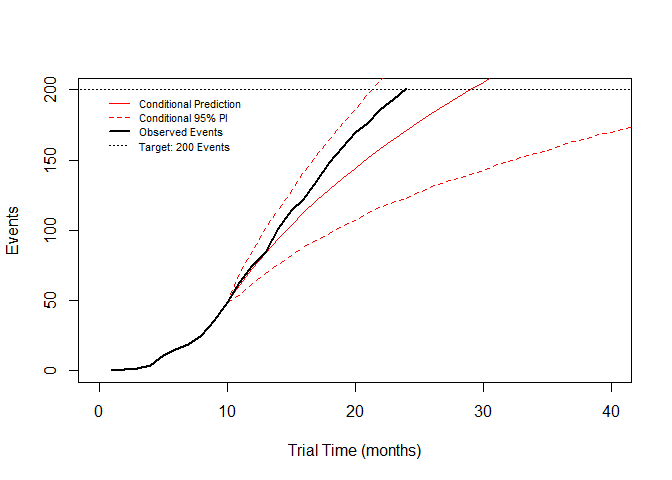The plot shows a reasonable agreement between the observed trajectory and the estimated conditional trajectory, which predicts 200 events would be reached by month 29. However, the 95% prediction interval is wide (particularly on the lower bound), and the prediction interval for the time to reach 200 events is 22-55 months. In practice therefore, event prediction at this time point is not that useful for estimating a trial end date.

We can now revisit the event prediction at two later time points (14 and 18 months), and compare the prediction accuracies:

trial_less_short <- set_assess_time(data=trial_long,time=14,detailed_output = FALSE)
predictions2 <- event_prediction(data=trial_less_short, Event="Censored",censoringOne=TRUE, type="Weibull", rcurve=recruit,max_time=60, cond_Events=101,cond_NatRisk=399,cond_Time=14, units="Months")

trial_mature <- set_assess_time(data=trial_long,time=18,detailed_output = FALSE)
predictions3 <- event_prediction(data=trial_mature, Event="Censored",censoringOne=TRUE, type="Weibull", rcurve=recruit,max_time=60, cond_Events=148,cond_NatRisk=352,cond_Time=18, units="Months")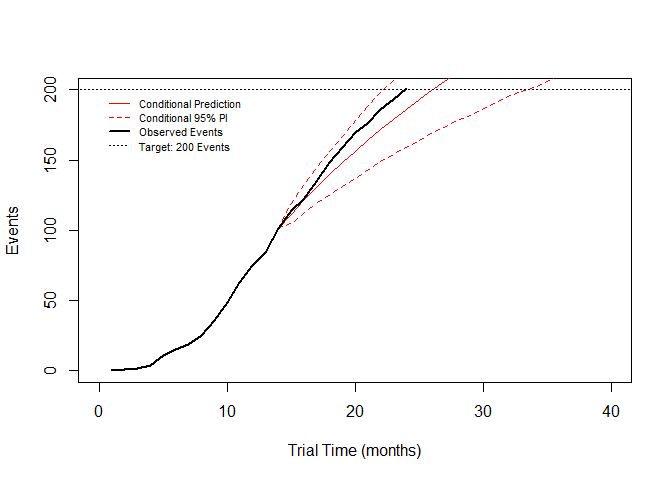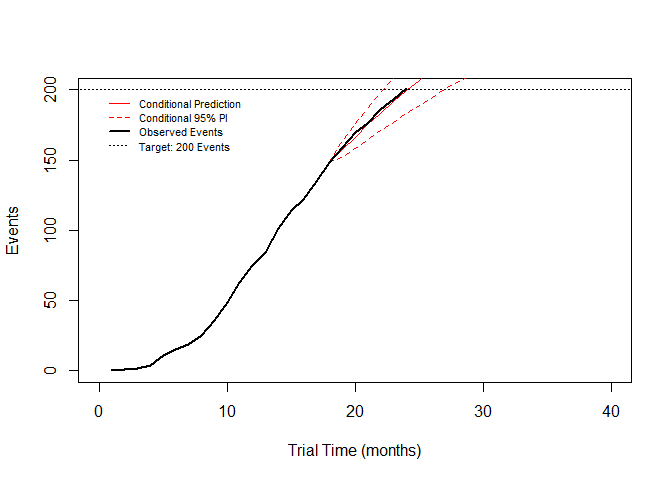Only 4 months later, the 95% prediction interval is down to 23-34 months, with an estimate of 27 months, while by 18 months, a tight 95% prediction interval of 23-27 months is possible around an estimate of 25 months. The tightening of the prediction interval over time is partly due to a reduction in the number of events remaining to be predicted, but also the increasing precision with which the parameters of the parametric Kaplan Meier curve fit. As a rule of thumb, 100 observed events appear to be required to give reasonable precision of estimation of Weibull parameters, but the greater the length of extrapolation required, the more precise the estimates have to be to give useful accuracy.

The conditional prediction interval can therefore demonstrate that in this case where a target of 200 events is desired, event prediction is probably too inaccurate to be of use at 10 months, somewhat indicative at 14 months, and fairly accurate by 18 months. As the trial has ‘progressed’, the estimate of the time of finishing has dropped from 29 months to 27 months and then again to 25 months. Depending on tolerance for risk, it may be more useful to use a smaller prediction interval e.g. 80 or 90%.

It should be noted that all of these prediction intervals are dependent upon the underlying distribution being Weibull, and that the same distribution applies to all patients, irrespective of e.g. the time of recruitment. They also depend on the recruitment distribution being fixed, i.e. containing no variability - this may be unreasonable for predictions at a very early time point. These limitations will tend to lead to the interval being narrower than it ought. Conversely, the prediction intervals do not account for knowledge obtained from prior trials or beliefs from the trial design stage; if there are strong prior expectations aligned with the observed data then the prediction intervals may be wider than they need be.

Finally, we can check the quality of the curve fitting and the degree of uncertainty around it.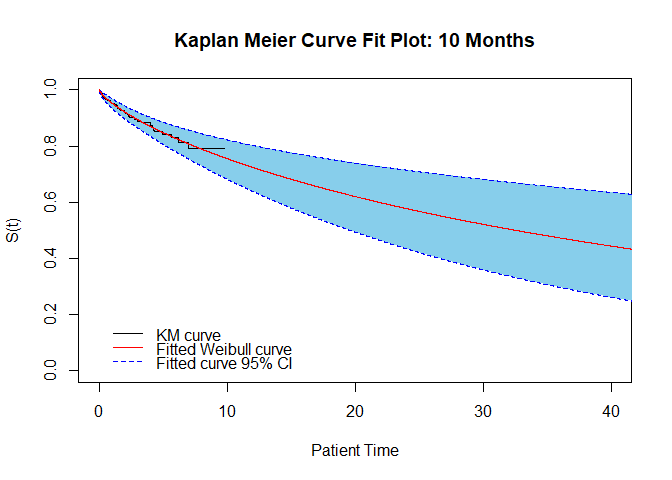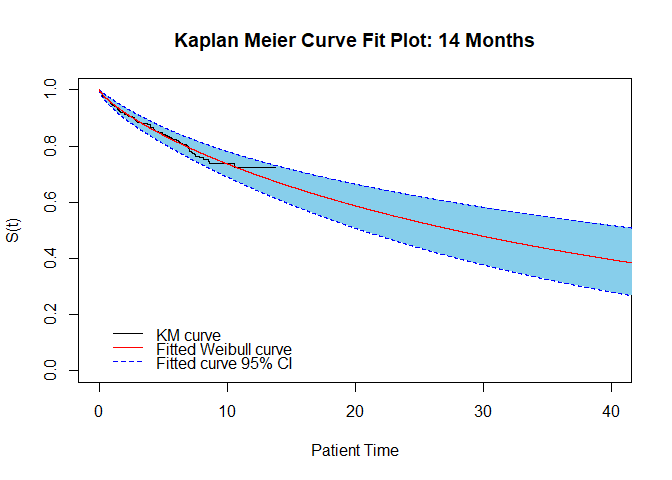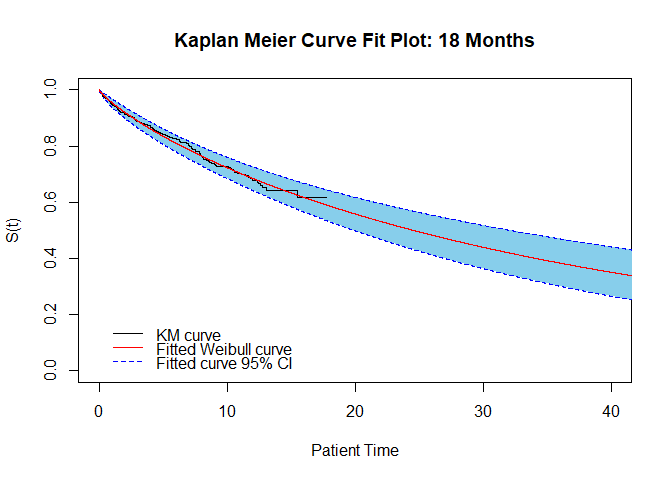From visual inspection, in all three cases there is reasonable agreement between the KM and fitted curves (albeit there is not much data at 10 months). This ensures their predictions are likely to at least be plausible. The width of the confidence interval using 10 months data is roughly double that for 18 months data. It is clear that the 10-month time point gives very little confidence about the Survival function when extrapolating. However, the 14 and 18 month time points provide some information about likely future behaviour, so do appear to be potentially useful for event prediction as long as the assumption of a Weibull distribution is reasonable.